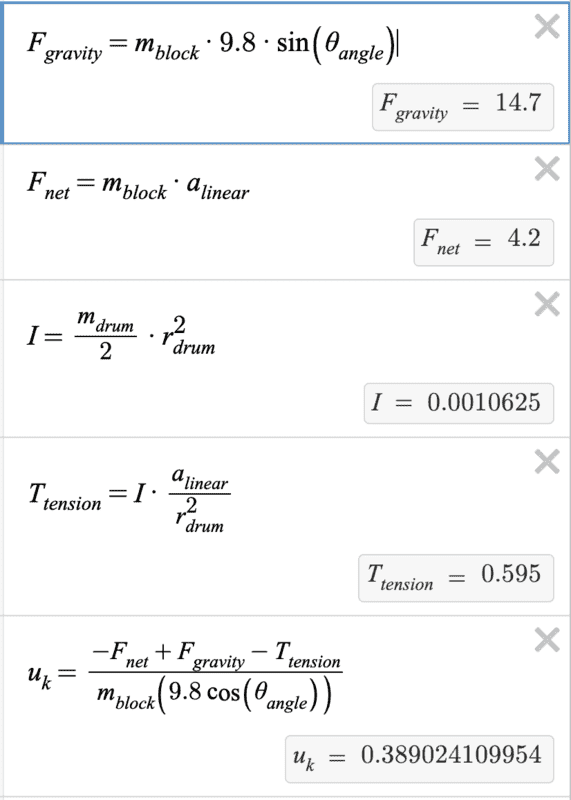# I don't understand how a tension related to a torque would have this formula

KittyCat1534
Homework Statement:
So I have a question which goes like this:

A 3.0 kg block rests on a 30° slope and is attached by a string of negligible mass to a solid drum of mass 0.85 kg and radius 5.0 cm, as shown in Fig. 10.29. When released, the block accelerates down the slope at 1.4 m/s2. What is the coefficient of friction between block and slope?

I've attached the diagram below. I've managed to find the answer, but I don't understand why the tension coming from the torque is T = IA/R^2, instead of just T = IA.

Thanks for the help!
Relevant Equations:
T = IA / R^2#### Attachments

Homework Helper
Gold Member
2022 Award
For one thing, T=Ia would be dimensionally wrong.

If the tension is T and the drum has radius r, what torque does the tension exert on the drum?
What equation relates the torque to I and to the angular acceleration, α?
What equation relates α to the radius and to the linear acceleration ?
Can you combine those to get the equation that puzzles you?

KittyCat1534
For one thing, T=Ia would be dimensionally wrong.

If the tension is T and the drum has radius r, what torque does the tension exert on the drum?
What equation relates the torque to I and to the angular acceleration, α?
What equation relates α to the radius and to the linear acceleration ?
Can you combine those to get the equation that puzzles you?
@haruspex
I'm sorry for the late reply, I believe that the equation which relates the torque to I and the angular acceleration is T = Ia. And since the linear acceleration is that of a tangent to the drum, linear acceleration = a/r. I combine those to get the equation T = (Ia)/r. Is this correct?

Homework Helper
Gold Member
2022 Award
the equation which relates the torque to I and the angular acceleration is T = Ia.
In standard use of variable names, that would be ##\tau=I\alpha##.
In the equation you queried, ##T=\frac{Ia}{r^2}##, T is tension, not torque, and a is linear acceleration, not angular acceleration.

Dimensionally,
[T]=MLT-2
[τ]=ML2T-2
[a]=LT-2
[α]=T-2
[r]=L
[ I]=MR2
Use those to check the equations in post #1.

linear acceleration = a/r
Here you are using a to mean angular acceleration, right? So in the above you are saying ##a=\frac{\alpha}r##? No, that's backwards: ##a=r\alpha ##.